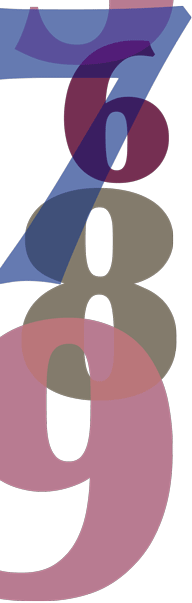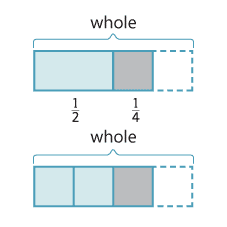# Year 7

## Number and Algebra

### Addition and subtraction of fractions

A facility with equivalent fractions helps students operate on them using addition and subtraction. At this level we work mostly with fractions with related denominators. In simple cases, fractions are related if the denominator of one is a multiple of the other. We use equivalent fractions to add fractions with different denominators. We can show $$\dfrac{1}{2}+\dfrac{1}{4}$$ using a rectangle. We can draw the same diagram differently to show that one-half plus one-quarter equals three-quarters.Detailed description

\begin{align}\frac{1}{2}+\frac{1}{4} &= \frac{2}{4} + \frac{1}{4} \text{(convert each to equivalent fraction with the same denominator)} \\&= \frac{3}{4}\end{align}